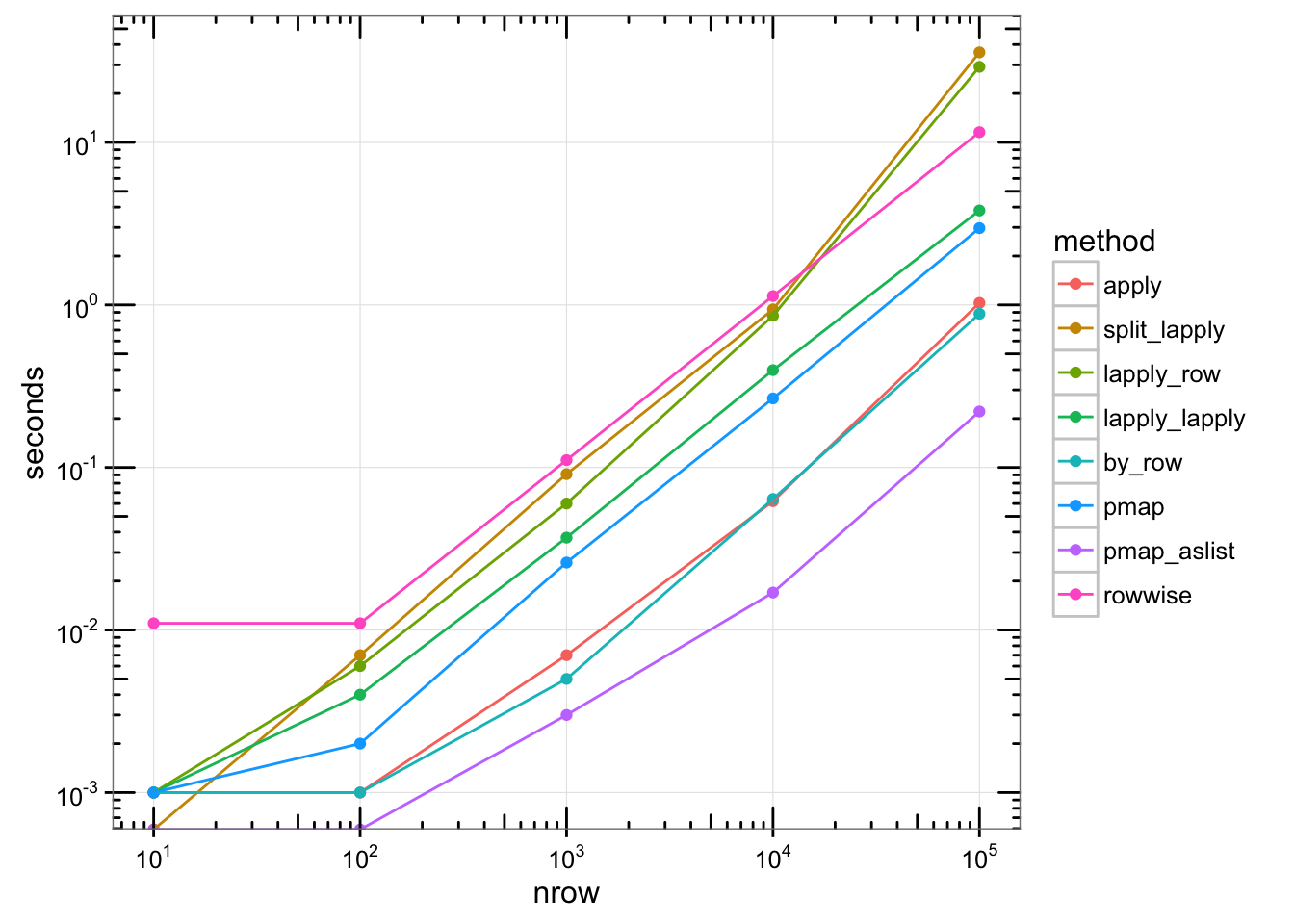Source for this document.

@dattali asked, “what’s a safe way to iterate over rows of a data frame?” The example was to convert each row into a list and return a list of lists, indexed first by column, then by row.

A number of people gave suggestions on Twitter, which I’ve collected here. I’ve benchmarked these methods with data of various sizes; scroll down to see a plot of times.

library(purrr)
library(dplyr)
library(tidyr)

# @dattali
# Using apply (only safe when all cols are same type)
f_apply <- function(df) {
apply(df, 1, function(row) as.list(row))
}

# @drob
# split + lapply
f_split_lapply <- function(df) {
df <- split(df, seq_len(nrow(df)))
lapply(df, function(row) as.list(row))
}

# @winston_chang
# lapply over row indices
f_lapply_row <- function(df) {
lapply(seq_len(nrow(df)), function(i) as.list(df[i,,drop=FALSE]))
}

# @winston_chang
# lapply + lapply: Treat data frame as list, and the slice out lists
f_lapply_lapply <- function(df) {
cols <- seq_len(length(df))
names(cols) <- names(df)

lapply(seq_len(nrow(df)), function(row) {
lapply(cols, function(col) {
df[[col]][[row]]
})
})
}

# @winston_chang
# purrr::by_row
f_by_row <- function(df) {
res <- by_row(df, function(row) as.list(row))
res$.out } # @JennyBryan # purrr::pmap f_pmap <- function(df) { pmap(df, list) } # purrr::pmap, but coerce df to a list first f_pmap_aslist <- function(df) { pmap(as.list(df), list) } # @krlmlr # dplyr::rowwise f_rowwise <- function(df) { df %>% rowwise %>% do(row = as.list(.)) } Benchmark each of them, using data sets with varying numbers of rows: run_benchmark <- function(nrow) { # Make some data df <- data.frame( x = rnorm(nrow), y = runif(nrow), z = runif(nrow) ) res <- list( apply = system.time(f_apply(df)), split_lapply = system.time(f_split_lapply(df)), lapply_row = system.time(f_lapply_row(df)), lapply_lapply = system.time(f_lapply_lapply(df)), by_row = system.time(f_by_row(df)), pmap = system.time(f_pmap(df)), pmap_aslist = system.time(f_pmap_aslist(df)), rowwise = system.time(f_rowwise(df)) ) # Get elapsed times res <- lapply(res, [[, "elapsed") # Add nrow to front res <- c(nrow = nrow, res) res } # Run the benchmarks for various size data all_times <- lapply(1:5, function(n) { run_benchmark(10^n) }) # Convert to data frame times <- lapply(all_times, as.data.frame) times <- do.call(rbind, times) knitr::kable(times) nrow apply split_lapply lapply_row lapply_lapply by_row pmap pmap_aslist rowwise 1e+01 0.001 0.000 0.001 0.001 0.001 0.001 0.000 0.011 1e+02 0.001 0.007 0.006 0.004 0.001 0.002 0.000 0.011 1e+03 0.007 0.091 0.060 0.037 0.005 0.026 0.003 0.111 1e+04 0.062 0.939 0.858 0.397 0.064 0.266 0.017 1.134 1e+05 1.029 35.802 29.170 3.811 0.882 2.969 0.221 11.556 ## Plot times This plot shows the number of seconds needed to process n rows, for each method. Both the x and y use log scales, so each step along the x scale represents a 10x increase in number of rows, and each step along the y scale represents a 10x increase in time. library(ggplot2) library(scales) # Convert to long format times_long <- gather(times, method, seconds, -nrow) # Set order of methods, for plots times_long$method <- factor(times_long\$method,
levels = c("apply", "split_lapply", "lapply_row", "lapply_lapply", "by_row",
"pmap", "pmap_aslist", "rowwise")
)

# Plot with log-log axes
ggplot(times_long, aes(x = nrow, y = seconds, colour = method)) +
geom_point() +
geom_line() +
annotation_logticks(sides = "trbl") +
theme_bw() +
scale_y_continuous(trans = log10_trans(),
breaks = trans_breaks("log10", function(x) 10^x),
labels = trans_format("log10", math_format(10^.x)),
minor_breaks = NULL) +
scale_x_continuous(trans = log10_trans(),
breaks = trans_breaks("log10", function(x) 10^x),
labels = trans_format("log10", math_format(10^.x)),
minor_breaks = NULL)